### 15.5.2.1 Steering the nonholonomic integrator

The main idea of the method can be clearly illustrated for the nonholonomic integrator,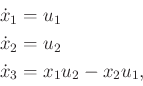(15.146)

which was considered throughout Section 15.5.1. This case will be explained in detail, and the methods obtained by generalizing the principles will subsequently be stated. The presentation given here is based on [727,846].

As was previously indicated, growing independent vector fields as quickly as possible is important. For the nonholonomic integrator,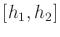, is linearly independent of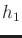and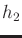, as observed in Example 15.12; thus, it satisfies this property. Consider steering the system from some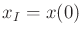to some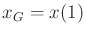while optimizing the cost functional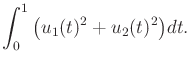(15.147)

The problem can be solved by using the constrained Lagrangian formulation, which was given in Section 13.4.3. The first step is to eliminate thevariables. From (15.146), the cost can be expressed in terms of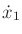and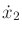by using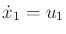and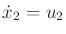. The third equation in (15.146) can be written as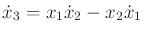(15.148)

and will be interpreted as a constraint on the Lagrangian, which is combined using a (scalar) Lagrange multiplier as explained in Section 13.4.3. Define the Lagrangian as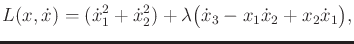(15.149)

in which the first term comes from the integrand of (15.147), and the second term comes from (15.148).

The Euler-Lagrange equation (13.118) yields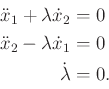(15.150)

Note that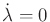implies that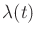is constant for all time. To obtain a differential equation that characterizes the optimal action trajectory, use the fact that for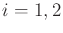,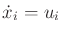and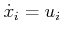. This yields the equations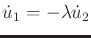and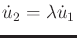. These can be represented as second-order linear differential equations. Based on its roots, the solution is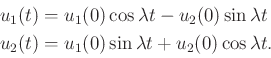(15.151)

Given initial and goal states, the optimal action trajectory is found by determining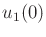,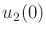, and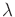. Suppose that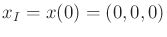and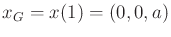for some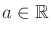. Other cases can be obtained by applying transformations in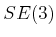to the solution.

The state trajectories for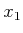and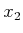can be obtained by integration of (15.151) because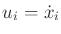for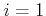and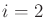. Starting from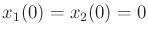, this yields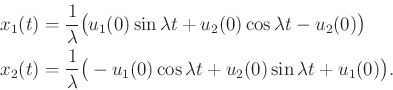(15.152)

To maintain the constraint that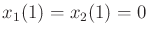,must be chosen as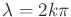for some integer. Integration of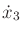yields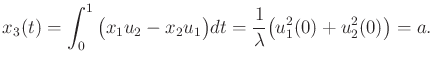(15.153)

The cost is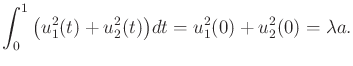(15.154)

The minimum cost is therefore achieved for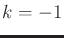, which yields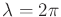and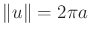. This fixes the magnitude of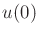, but any direction may be chosen.

The steering problem can be solved in two phases:

1. Apply any action trajectory to steerandto their desired values while neglecting to consider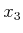.
2. Apply the solution just developed to steerto the goal whileandreturn to their values obtained in the first phase.
This idea can be generalized to other systems.

Steven M LaValle 2020-08-14